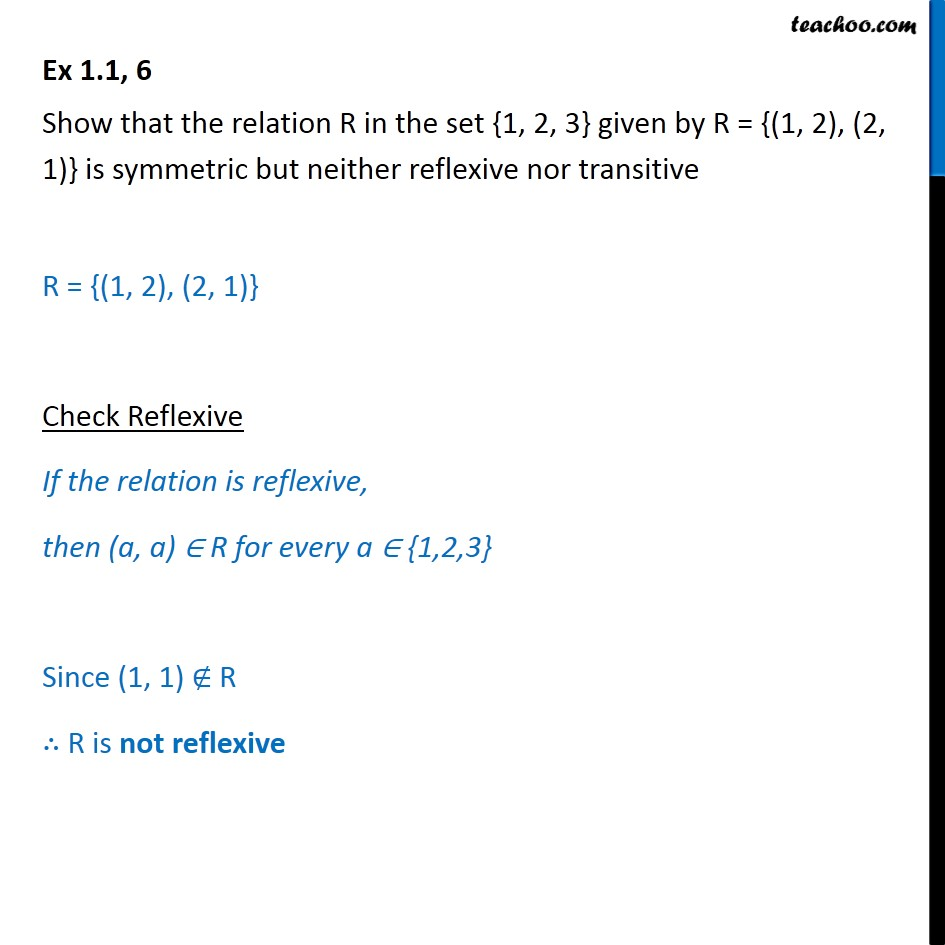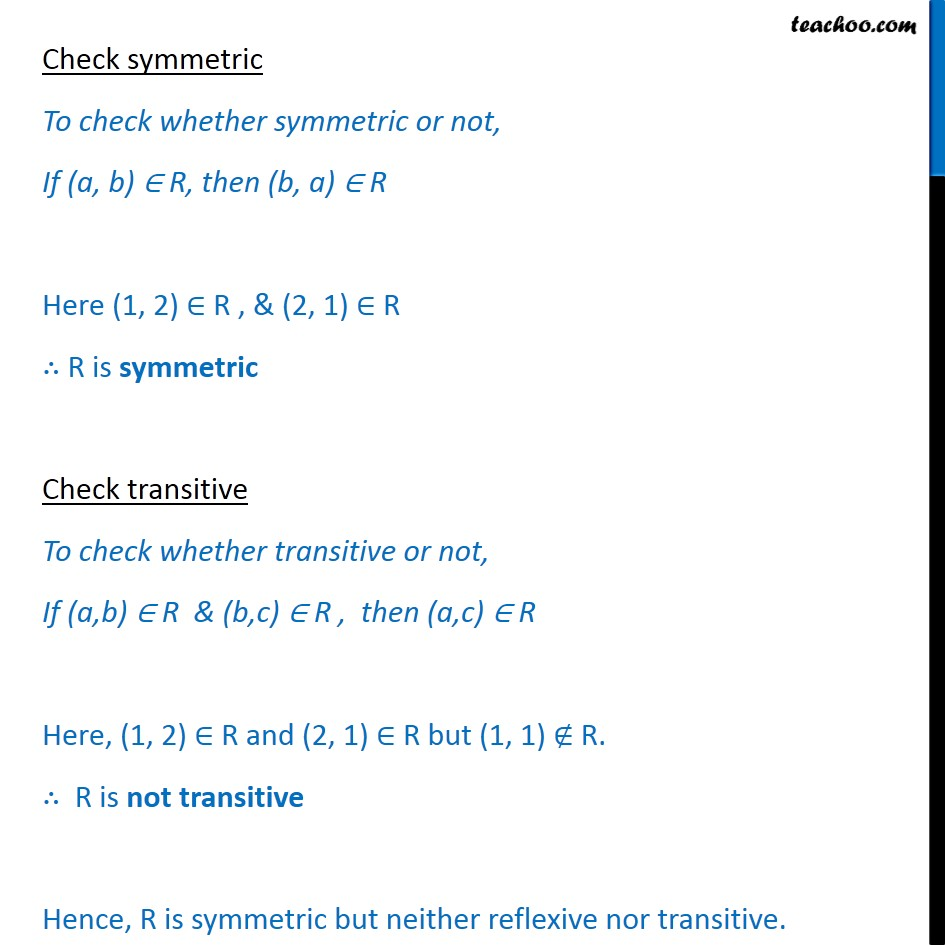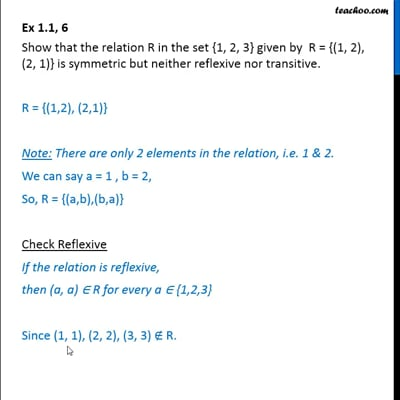Ex 1.1

Chapter 1 Class 12 Relation and Functions
Serial order wiseThis video is only available for Teachoo black users

Introducing your new favourite teacher - Teachoo Black, at only ₹83 per month

### Transcript

Ex 1.1, 6 Show that the relation R in the set {1, 2, 3} given by R = {(1, 2), (2, 1)} is symmetric but neither reflexive nor transitive R = {(1, 2), (2, 1)} Check Reflexive If the relation is reflexive, then (a, a) R for every a {1,2,3} Since (1, 1) R R is not reflexive Check symmetric To check whether symmetric or not, If (a, b) R, then (b, a) R Here (1, 2) R , & (2, 1) R R is symmetric Check transitive To check whether transitive or not, If (a,b) R & (b,c) R , then (a,c) R Here, (1, 2) R and (2, 1) R but (1, 1) R. R is not transitive Hence, R is symmetric but neither reflexive nor transitive.